﻿ 分数阶电报方程一类有效的普遍性差分方法 A Kind of Efficient Universal Differential Methods for Fractional Telegraph Equations

Vol. 08  No. 09 ( 2019 ), Article ID: 32138 , 12 pages
10.12677/AAM.2019.89181

A Kind of Efficient Universal Differential Methods for Fractional Telegraph Equations

Lifei Wu, Xiaozhong Yang*

School of Mathematics and Physics, North China Electric Power University, BeijingReceived: Aug. 21st, 2019; accepted: Sep. 5th, 2019; published: Sep. 12th, 2019ABSTRACT

A kind of universal difference method is constructed for the time fractional telegraph equation. The stability and convergence of the difference method are analyzed by Fourier method. Finally, the effectiveness of the method for solving the fractional telegraph equation is verified by numerical experiments. The comparison of different θ values is carried out. The numerical results show that the numerical solution is better when θ is around 0.5. Therefore, it is effective to solve the time-fractional telegraph equation by the universal difference method.

Keywords:Time Fractional Telegraph Equation, Universal Difference Method, Fourier Method, Stability, Numerical Experiment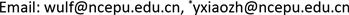1. 引言

2. 分数阶电报方程的普遍性差分格式

2.1. 分数阶电报方程

$\frac{{\partial }^{2\alpha }u\left(x,t\right)}{\partial {t}^{2\alpha }}+\frac{{\partial }^{\alpha }u\left(x,t\right)}{\partial {t}^{\alpha }}=k\frac{{\partial }^{2}u\left(x,t\right)}{\partial {x}^{2}}+f\left(x,t\right),\text{\hspace{0.17em}}\text{\hspace{0.17em}}\text{0}0,\text{\hspace{0.17em}}\frac{\text{1}}{\text{2}}<\alpha \le 1$ (1)

2.2. 普遍性差分格式的构造

$\frac{{\partial }^{\alpha }u\left({x}_{i},{t}_{k+1}\right)}{\partial {t}^{\alpha }}=\frac{{\tau }^{-\alpha }}{\Gamma \left(2-\alpha \right)}\underset{j=0}{\overset{k}{\sum }}{c}_{j}{\nabla }_{t}u\left({x}_{i},{t}_{k-j}\right)+o\left({\tau }^{2-\alpha }\right)$ (2)

$\frac{{\partial }^{2\alpha }u\left({x}_{i},{t}_{k+1}\right)}{\partial {t}^{2\alpha }}=\frac{{\tau }^{-2\alpha }}{\Gamma \left(3-2\alpha \right)}\underset{j=0}{\overset{k\text{-}1}{\sum }}{d}_{j}{\delta }_{t}^{2}u\left({x}_{i},{t}_{k-j}\right)+2{d}_{k}\left(u\left({x}_{i},{t}_{1}\right)-u\left({x}_{i},{t}_{0}\right)-\tau {u}_{t}\left({x}_{i}\right)\right)+o\left({\tau }^{3-2\alpha }\right)$ (3)

$j=0$ 或者 $j=k$ 时上式会出现项 ${u}_{i}^{-1}$ ，用初始边界条件去逼近 ${u}_{i}^{-1}$${u}_{i}^{-1}={u}_{i}^{1}-2k{u}_{1}\left({x}_{i}\right)+O\left({\tau }^{3}\right)$ ，误差忽略不计。

$\frac{{\partial }^{2}u\left({x}_{i},{t}_{k+1}\right)}{\partial {x}^{2}}={\delta }_{x}^{2}\left[\theta u\left({x}_{i},{t}_{k+1}\right)+\left(1-\theta \right)u\left({x}_{i},{t}_{k}\right)\right]+o\left({h}^{2}\right)$ (4)

$\frac{{\tau }^{-2\alpha }}{\Gamma \left(3-2\alpha \right)}\underset{j=0}{\overset{k\text{-}1}{\sum }}{c}_{j}{\delta }_{t}^{2}u\left({x}_{i},{t}_{k-j}\right)+\frac{{\tau }^{-\alpha }}{\Gamma \left(2-\alpha \right)}\underset{j=0}{\overset{k}{\sum }}{d}_{j}{\nabla }_{t}u\left({x}_{i},{t}_{k-j}\right)=\frac{k}{{h}^{2}}{\delta }_{x}^{2}\left[\theta u\left({x}_{i},{t}_{k+1}\right)+\left(1-\theta \right)u\left({x}_{i},{t}_{k}\right)\right]+f\left({x}_{i},{t}_{k+1}\right)$ (5)

$a\underset{j=0}{\overset{k-1}{\sum }}{d}_{j}{\delta }_{t}^{2}{u}_{i}^{k-j}+2{d}_{k}\left[u\left({x}_{i},{t}_{1}\right)-u\left({x}_{i},{t}_{0}\right)-\tau {u}_{t}\left({x}_{i}\right)\right]+b\underset{j=0}{\overset{k-1}{\sum }}{c}_{j}{\nabla }_{t}{u}_{i}^{k-j}=r{\delta }_{x}^{2}\left(\theta {u}_{i}^{k+1}+\left(1-\theta \right){u}_{i}^{k}\right)+{f}_{i}^{k+1}.$

$\left\{\begin{array}{l}\left[\left(2a+b\right)E+r\theta G\right]{U}^{1}=\left[\left(2a+b\right)E-r\left(1-\theta \right)G\right]{U}^{0}+2a\tau H+{F}^{1},\\ A{U}^{k+1}=B{U}^{k}+{q}_{1}{U}^{k-1}+\cdots +{q}_{k-2}{U}^{2}+{q}_{k-1}{U}^{1}+{q}_{k}{U}^{0}+2a{d}_{k}\tau H+{F}^{k+1}.\end{array}$ (6)

$G=\left[\begin{array}{ccccc}2& -1& & & \\ -1& 2& -1& & \\ & & \ddots & \ddots & \ddots \\ & & \ddots & \ddots & -1\\ & & & -1& 2\end{array}\right].$

3. 普遍性差分格式解的稳定性和收敛性分析

3.1. 差分格式稳定性分析

$\left\{\begin{array}{l}\left(2a+b+2r\theta \right){\epsilon }_{i}^{1}-r\theta \left({\epsilon }_{i+1}^{1}+{\epsilon }_{i-1}^{1}\right)=\left(2a+b-2r\left(1-\theta \right)\right){\epsilon }_{i}^{0}+\left(1-\theta \right)r\left({\epsilon }_{i+1}^{0}+{\epsilon }_{i-1}^{0}\right),\\ \left(a+b+2r\theta \right){\epsilon }_{i}^{k+1}-r\theta \left({\epsilon }_{i+1}^{k+1}+{\epsilon }_{i-1}^{k+1}\right)=\left[a\left(2{d}_{0}-{d}_{1}\right)+b\left({c}_{0}-{c}_{1}\right)-2r\left(1-\theta \right)\right]{\epsilon }_{i}^{k}\\ \text{\hspace{0.17em}}\text{\hspace{0.17em}}\text{\hspace{0.17em}}\text{\hspace{0.17em}}\text{\hspace{0.17em}}\text{\hspace{0.17em}}\text{\hspace{0.17em}}\text{\hspace{0.17em}}\text{\hspace{0.17em}}\text{\hspace{0.17em}}\text{\hspace{0.17em}}\text{\hspace{0.17em}}\text{\hspace{0.17em}}\text{\hspace{0.17em}}\text{\hspace{0.17em}}\text{\hspace{0.17em}}\text{\hspace{0.17em}}\text{\hspace{0.17em}}\text{\hspace{0.17em}}\text{\hspace{0.17em}}\text{\hspace{0.17em}}\text{\hspace{0.17em}}\text{\hspace{0.17em}}\text{\hspace{0.17em}}\text{\hspace{0.17em}}\text{\hspace{0.17em}}\text{\hspace{0.17em}}\text{\hspace{0.17em}}\text{\hspace{0.17em}}\text{\hspace{0.17em}}\text{\hspace{0.17em}}\text{\hspace{0.17em}}\text{\hspace{0.17em}}\text{\hspace{0.17em}}\text{\hspace{0.17em}}\text{\hspace{0.17em}}\text{\hspace{0.17em}}\text{\hspace{0.17em}}\text{\hspace{0.17em}}\text{\hspace{0.17em}}\text{\hspace{0.17em}}\text{\hspace{0.17em}}\text{\hspace{0.17em}}\text{\hspace{0.17em}}\text{\hspace{0.17em}}\text{\hspace{0.17em}}\text{\hspace{0.17em}}\text{\hspace{0.17em}}\text{\hspace{0.17em}}+r\left(1-\theta \right)\left({\epsilon }_{i+1}^{k}+{\epsilon }_{i-1}^{k}\right)+\underset{j=1}{\overset{k-1}{\sum }}{q}_{j}{\epsilon }_{i}^{k-j}+{q}_{k}{\epsilon }_{i}^{0}.\end{array}$ (7)

${\epsilon }^{n}\left(x\right)=\left\{\begin{array}{l}{\epsilon }_{l}^{n},x\in \left({x}_{l-1/2},{x}_{l+1/2}\right],l=1,2,3,\cdots ,M-1,\\ 0,x\in \left[{L}_{1},h/2\right]\cup \left({L}_{2}-h/2,{L}_{2}\right].\end{array}$

${\epsilon }^{n}\left(x\right)$ 进行傅立叶展开：

${\epsilon }^{n}\left(x\right)=\underset{j=-\infty }{\overset{+\infty }{\sum }}{v}_{j}^{n}{\text{e}}^{i\frac{2j\pi x}{L}}\left(i=\sqrt{-1}\right),n=0,1,2,\cdots ,N$

${‖{\epsilon }^{n}\left(x\right)‖}^{2}=\underset{j=1}{\overset{M-1}{\sum }}h{|{\epsilon }_{j}^{n}|}^{2}=L\underset{j=-\infty }{\overset{+\infty }{\sum }}{|{v}_{j}^{n}|}^{2},n=0,1,\cdots ,N$

$k=1$ 时，

$\left(2a+b+2r\theta -r\theta {\text{e}}^{i\lambda h}-r\theta {\text{e}}^{-i\lambda h}\right){v}^{1}=\left(2a+b-2r\left(1-\theta \right)+\left(1-\theta \right)r\left({\text{e}}^{i\lambda h}+{\text{e}}^{-i\lambda h}\right)\right){v}^{0}$ (8)

${\text{e}}^{±i\lambda h}=\mathrm{cos}\lambda h±i\mathrm{sin}\lambda h$${\mathrm{sin}}^{2}\frac{\lambda h}{2}=-\frac{1}{4}\left({\text{e}}^{i\lambda h}-2+{\text{e}}^{-i\lambda h}\right)$ ，可得

$|{v}^{1}|=\frac{|\text{2}a+b-4r\left(1-\theta \right){\mathrm{sin}}^{2}\frac{\lambda h}{2}|}{|2a+b+4r\theta {\mathrm{sin}}^{2}\lambda h|}|{v}^{0}|.$

$\frac{|\text{2}a+b-4r\left(1-\theta \right){\mathrm{sin}}^{2}\frac{\lambda h}{2}|}{|2a+b+4r\theta {\mathrm{sin}}^{2}\lambda h|}\le 1$ ，可得 $2r\left(1-2\theta \right)\le 2a+b$ ；因此，普遍性差分格式的稳定条件为：当 $0\le \theta <\frac{1}{2}$ 时， $r\le \frac{2a+b}{2\left(1-2\theta \right)}$ ；当 $\frac{1}{2}\le \theta \le 1$ 时，无条件稳定。

$k>1$ 时，

$\begin{array}{l}\left(a+b+2r\theta -r\theta \left({\text{e}}^{i\lambda h}+{\text{e}}^{-i\lambda h}\right)\right){v}^{k+1}\\ =\left[a\left(2{d}_{0}-{d}_{1}\right)+b\left({c}_{0}-{c}_{1}\right)-2r\left(1-\theta \right)+r\left(1-\theta \right)\left({\text{e}}^{i\lambda h}+{\text{e}}^{-i\lambda h}\right)\right]{v}^{k}+\underset{j=1}{\overset{k-1}{\sum }}{q}_{j}{v}^{k-j}+{q}_{k}{v}^{0}\end{array}$ (9)

$\begin{array}{l}\left(a+b+4r\theta {\mathrm{sin}}^{2}\lambda h\right)|{v}^{k+1}|\\ =\left[a\left(2{d}_{0}-{d}_{1}\right)+b\left({c}_{0}-{c}_{1}\right)-4r\left(1-\theta \right){\mathrm{sin}}^{2}\lambda h\right]|{v}^{k}|+\underset{j=1}{\overset{k-1}{\sum }}{q}_{j}|{v}^{k-j}|+{q}_{k}|{v}^{0}|\\ \le \left\{|\left[a\left(2{d}_{0}-{d}_{1}\right)+b\left({c}_{0}-{c}_{1}\right)-4r\left(1-\theta \right){\mathrm{sin}}^{2}\lambda h\right]|+\underset{j=1}{\overset{k-1}{\sum }}|{q}_{j}|+|{q}_{k}|\right\}|{v}^{0}|\\ \le \left\{|\left[a\left(2{d}_{0}-{d}_{1}\right)+b\left({c}_{0}-{c}_{1}\right)-4r\left(1-\theta \right){\mathrm{sin}}^{2}\lambda h\right]|+b{c}_{1}+a\left({d}_{0}-{d}_{1}\right)\right\}|{v}^{0}|\\ \le |\left[a+b-4r\left(1-\theta \right){\mathrm{sin}}^{2}\lambda h\right]||{v}^{0}|\end{array}$

$\frac{|a+b-4r\left(1-\theta \right){\mathrm{sin}}^{2}\frac{\lambda h}{2}|}{|a+b+4r\theta {\mathrm{sin}}^{2}\lambda h|}\le 1$ ，可得 $2r\left(1-2\theta \right)\le 2a+b$ ；此时普遍性差分格式的稳定条件为：当 $0\le \theta <\frac{1}{2}$ 时， $r\le \frac{a+b}{2\left(1-2\theta \right)}$ ；当 $\frac{1}{2}\le \theta \le 1$ 时，无条件稳定。

3.2. 差分格式收敛性分析

${e}_{j}^{k}=u\left({x}_{j},{t}_{k}\right)-{u}_{j}^{k}\text{\hspace{0.17em}}\left(k=1,2,\cdots ,N;j=1,2,\cdots ,M-1\right)$

${e}^{k}={\left[{e}_{1}^{k},{e}_{2}^{k},\cdots ,{e}_{M-1}^{k}\right]}^{\text{T}}$${R}^{k}={\left[{R}_{1}^{k},{R}_{2}^{k},\cdots ,{R}_{M-1}^{k}\right]}^{\text{T}}.$

$\begin{array}{l}{e}_{i}^{0}=0,i=0,1,\cdots ,M,\\ {e}_{0}^{k}={e}_{M}^{k}=0,k=0,1,\cdots ,N\end{array}$

${e}^{k}\left(x\right)=\left\{\begin{array}{l}0,0\le x\le \frac{h}{2},\\ {e}_{j}^{k},{x}_{j}-\frac{h}{2}

${R}^{k}\left(x\right)=\left\{\begin{array}{l}0,0\le x\le \frac{h}{2},\\ {R}_{j}^{k},{x}_{j}-\frac{h}{2}

${e}^{k}\left(x\right),{R}^{k}\left(x\right)$ 的Fourier展开形式为：

${e}^{k}\left(x\right)=\underset{l=-\infty }{\overset{\infty }{\sum }}{\eta }_{k}\left(l\right){\text{e}}^{i2\pi lx/L}$ ，其中系数 ${\eta }_{k}\left(l\right)=\frac{1}{L}{\int }_{0}^{L}{e}^{k}\left(x\right){\text{e}}^{-i2\pi lx/L}\text{d}x.$

${R}^{k}\left(x\right)=\underset{l=-\infty }{\overset{\infty }{\sum }}{\xi }_{k}\left(l\right){\text{e}}^{i2\pi lx/L}$ ，其中系数 ${\xi }_{k}\left(l\right)=\frac{1}{L}{\int }_{0}^{L}{R}^{k}\left(x\right){\text{e}}^{-i2\pi lx/L}\text{d}x.$

${‖{e}^{k}‖}_{2}^{2}=\underset{j=1}{\overset{M-1}{\sum }}h{|{e}_{j}^{k}|}^{2}=\underset{l=-\infty }{\overset{\infty }{\sum }}{|{\eta }_{k}\left(l\right)|}^{2},$ (10)

${‖{R}^{k}‖}_{2}^{2}=\underset{j=1}{\overset{M-1}{\sum }}h{|{R}_{j}^{k}|}^{2}=\underset{l=-\infty }{\overset{\infty }{\sum }}{|{\xi }_{k}\left(l\right)|}^{2}.$ (11)

$\left\{\begin{array}{l}\left(2a+b+2r\theta \right){e}_{i}^{1}-r\theta \left({e}_{i+1}^{1}+{e}_{i-1}^{1}\right)=\left(2a+b-2r\left(1-\theta \right)\right){e}_{i}^{0}+\left(1-\theta \right)r\left({e}_{i+1}^{0}+{e}_{i-1}^{0}\right)+{R}_{i}^{1},\\ \left(a+b+2r\theta \right){e}_{i}^{k+1}-r\theta \left({e}_{i+1}^{k+1}+{e}_{i-1}^{k+1}\right)=\left[a\left(2{d}_{0}-{d}_{1}\right)+b\left({c}_{0}-{c}_{1}\right)-2r\left(1-\theta \right)\right]{e}_{i}^{k}\\ \text{\hspace{0.17em}}\text{\hspace{0.17em}}\text{\hspace{0.17em}}\text{\hspace{0.17em}}\text{\hspace{0.17em}}\text{\hspace{0.17em}}\text{\hspace{0.17em}}\text{\hspace{0.17em}}\text{\hspace{0.17em}}\text{\hspace{0.17em}}\text{\hspace{0.17em}}\text{\hspace{0.17em}}\text{\hspace{0.17em}}\text{\hspace{0.17em}}\text{\hspace{0.17em}}\text{\hspace{0.17em}}\text{\hspace{0.17em}}\text{\hspace{0.17em}}\text{\hspace{0.17em}}\text{\hspace{0.17em}}\text{\hspace{0.17em}}\text{\hspace{0.17em}}\text{\hspace{0.17em}}\text{\hspace{0.17em}}\text{\hspace{0.17em}}\text{\hspace{0.17em}}\text{\hspace{0.17em}}\text{\hspace{0.17em}}\text{\hspace{0.17em}}\text{\hspace{0.17em}}\text{\hspace{0.17em}}\text{\hspace{0.17em}}\text{\hspace{0.17em}}\text{\hspace{0.17em}}\text{\hspace{0.17em}}\text{\hspace{0.17em}}\text{\hspace{0.17em}}\text{\hspace{0.17em}}\text{\hspace{0.17em}}\text{\hspace{0.17em}}\text{\hspace{0.17em}}\text{\hspace{0.17em}}\text{\hspace{0.17em}}\text{\hspace{0.17em}}\text{\hspace{0.17em}}\text{\hspace{0.17em}}\text{\hspace{0.17em}}\text{\hspace{0.17em}}+r\left(1-\theta \right)\left({e}_{i+1}^{k}+{e}_{i-1}^{k}\right)+\underset{j=1}{\overset{k-1}{\sum }}{q}_{j}{e}_{i}^{k-j}+{q}_{k}{e}_{i}^{0}+{R}_{i}^{k+1}.\end{array}$ (12)

$|{\eta }_{k}|\le {c}_{2}{a}_{k-1}^{-1}{\xi }_{1},k=1,2,\cdots ,N.$

${‖{R}^{k}‖}_{2}\le {c}_{1}\sqrt{L}\left({\tau }^{2-\alpha }+{h}^{2}\right),$ (13)

(11)式等式右边的系数数列是收敛的，因此存在一个正数 ${c}_{2}$ 使得

$|{\xi }_{k}|\equiv |{\xi }_{k}\left(m\right)|\le {c}_{2}{\xi }_{1}\equiv {c}_{2}|{\xi }_{1}\left(m\right)|,k=1,2,\cdots ,N.$ (14)

$|{\xi }_{k}|\le {c}_{2}{\xi }_{1},k=1,2,\cdots ,N.$

$k=1$ 时，有

$\left(2a+b+2r\theta \right){e}_{i}^{1}-r\theta \left({e}_{i+1}^{1}+{e}_{i-1}^{1}\right)=\left(2a+b-2r\left(1-\theta \right)\right){e}_{i}^{0}+\left(1-\theta \right)r\left({e}_{i+1}^{0}+{e}_{i-1}^{0}\right)+{R}_{i}^{1},$

$|{\eta }_{1}|=\frac{1}{|2a+b+4r\theta {\mathrm{sin}}^{2}\lambda h|}{\xi }_{1}\le {q}_{0}^{-1}{\xi }_{1}.$

$k>1$ 时，

$\begin{array}{l}\left(a+b+2r\theta \right){e}_{i}^{k+1}-r\theta \left({e}_{i+1}^{k+1}+{e}_{i-1}^{k+1}\right)\\ =\left[a\left(2{d}_{0}-{d}_{1}\right)+b\left({c}_{0}-{c}_{1}\right)-2r\left(1-\theta \right)\right]{e}_{i}^{k}+r\left(1-\theta \right)\left({e}_{i+1}^{k}+{e}_{i-1}^{k}\right)+\underset{j=1}{\overset{k-1}{\sum }}{q}_{j}{e}_{i}^{k-j}+{q}_{k}{e}_{i}^{0}+{R}_{i}^{k+1}\end{array}$

$\begin{array}{l}\left(a+b+4r\theta {\mathrm{sin}}^{2}\lambda h\right)|{\eta }^{k+1}|=\left[a\left(2{d}_{0}-{d}_{1}\right)+b\left({c}_{0}-{c}_{1}\right)-4r\left(1-\theta \right){\mathrm{sin}}^{2}\lambda h\right]|{\eta }^{k}|+\underset{j=1}{\overset{k-1}{\sum }}{q}_{j}|{\eta }^{k-j}|+|{R}^{k}|\\ \text{\hspace{0.17em}}\text{\hspace{0.17em}}\text{\hspace{0.17em}}\text{\hspace{0.17em}}\text{\hspace{0.17em}}\text{\hspace{0.17em}}\text{\hspace{0.17em}}\text{\hspace{0.17em}}\text{\hspace{0.17em}}\text{\hspace{0.17em}}\text{\hspace{0.17em}}\text{\hspace{0.17em}}\text{\hspace{0.17em}}\text{\hspace{0.17em}}\text{\hspace{0.17em}}\text{\hspace{0.17em}}\text{\hspace{0.17em}}\text{\hspace{0.17em}}\text{\hspace{0.17em}}\text{\hspace{0.17em}}\text{\hspace{0.17em}}\text{\hspace{0.17em}}\text{\hspace{0.17em}}\text{\hspace{0.17em}}\text{\hspace{0.17em}}\text{\hspace{0.17em}}\text{\hspace{0.17em}}\text{\hspace{0.17em}}\text{\hspace{0.17em}}\text{\hspace{0.17em}}\text{\hspace{0.17em}}\text{\hspace{0.17em}}\text{\hspace{0.17em}}\text{\hspace{0.17em}}\le \left\{|\left[a\left(2{d}_{0}-{d}_{1}\right)+b\left({c}_{0}-{c}_{1}\right)-4r\left(1-\theta \right){\mathrm{sin}}^{2}\lambda h\right]|{q}_{k}^{-1}+\underset{j=1}{\overset{k-1}{\sum }}|{q}_{j}|{q}_{k-j}^{-1}+1\right\}{c}_{2}|{\xi }_{1}|\\ \text{\hspace{0.17em}}\text{\hspace{0.17em}}\text{\hspace{0.17em}}\text{\hspace{0.17em}}\text{\hspace{0.17em}}\text{\hspace{0.17em}}\text{\hspace{0.17em}}\text{\hspace{0.17em}}\text{\hspace{0.17em}}\text{\hspace{0.17em}}\text{\hspace{0.17em}}\text{\hspace{0.17em}}\text{\hspace{0.17em}}\text{\hspace{0.17em}}\text{\hspace{0.17em}}\text{\hspace{0.17em}}\text{\hspace{0.17em}}\text{\hspace{0.17em}}\text{\hspace{0.17em}}\text{\hspace{0.17em}}\text{\hspace{0.17em}}\text{\hspace{0.17em}}\text{\hspace{0.17em}}\text{\hspace{0.17em}}\text{\hspace{0.17em}}\text{\hspace{0.17em}}\text{\hspace{0.17em}}\text{\hspace{0.17em}}\text{\hspace{0.17em}}\text{\hspace{0.17em}}\text{\hspace{0.17em}}\text{\hspace{0.17em}}\text{\hspace{0.17em}}\text{\hspace{0.17em}}\le \left\{|\left[a\left(2{d}_{0}-{d}_{1}\right)+b\left({c}_{0}-{c}_{1}\right)-4r\left(1-\theta \right){\mathrm{sin}}^{2}\lambda h\right]|+\underset{j=1}{\overset{k-1}{\sum }}|{q}_{j}|+{q}_{k}\right\}{c}_{2}{q}_{k}^{-1}|{\xi }_{1}|\\ \text{\hspace{0.17em}}\text{\hspace{0.17em}}\text{\hspace{0.17em}}\text{\hspace{0.17em}}\text{\hspace{0.17em}}\text{\hspace{0.17em}}\text{\hspace{0.17em}}\text{\hspace{0.17em}}\text{\hspace{0.17em}}\text{\hspace{0.17em}}\text{\hspace{0.17em}}\text{\hspace{0.17em}}\text{\hspace{0.17em}}\text{\hspace{0.17em}}\text{\hspace{0.17em}}\text{\hspace{0.17em}}\text{\hspace{0.17em}}\text{\hspace{0.17em}}\text{\hspace{0.17em}}\text{\hspace{0.17em}}\text{\hspace{0.17em}}\text{\hspace{0.17em}}\text{\hspace{0.17em}}\text{\hspace{0.17em}}\text{\hspace{0.17em}}\text{\hspace{0.17em}}\text{\hspace{0.17em}}\text{\hspace{0.17em}}\text{\hspace{0.17em}}\text{\hspace{0.17em}}\text{\hspace{0.17em}}\text{\hspace{0.17em}}\text{\hspace{0.17em}}\text{\hspace{0.17em}}\le \left\{|\left[a\left(2{d}_{0}-{d}_{1}\right)+b\left({c}_{0}-{c}_{1}\right)-4r\left(1-\theta \right){\mathrm{sin}}^{2}\lambda h\right]|+b{c}_{1}+a\left({d}_{0}-{d}_{1}\right)\right\}{c}_{2}{q}_{k}^{-1}|{\xi }_{1}|\\ \text{\hspace{0.17em}}\text{\hspace{0.17em}}\text{\hspace{0.17em}}\text{\hspace{0.17em}}\text{\hspace{0.17em}}\text{\hspace{0.17em}}\text{\hspace{0.17em}}\text{\hspace{0.17em}}\text{\hspace{0.17em}}\text{\hspace{0.17em}}\text{\hspace{0.17em}}\text{\hspace{0.17em}}\text{\hspace{0.17em}}\text{\hspace{0.17em}}\text{\hspace{0.17em}}\text{\hspace{0.17em}}\text{\hspace{0.17em}}\text{\hspace{0.17em}}\text{\hspace{0.17em}}\text{\hspace{0.17em}}\text{\hspace{0.17em}}\text{\hspace{0.17em}}\text{\hspace{0.17em}}\text{\hspace{0.17em}}\text{\hspace{0.17em}}\text{\hspace{0.17em}}\text{\hspace{0.17em}}\text{\hspace{0.17em}}\text{\hspace{0.17em}}\text{\hspace{0.17em}}\text{\hspace{0.17em}}\text{\hspace{0.17em}}\text{\hspace{0.17em}}\text{\hspace{0.17em}}\le |\left[a+b-4r\left(1-\theta \right){\mathrm{sin}}^{2}\lambda h\right]|{c}_{2}{q}_{k}^{-1}|{\xi }_{1}|\end{array}$

$|{\eta }^{k+1}|\le \frac{|a+b-4r\left(1-\theta \right){\mathrm{sin}}^{2}\lambda h|}{a+b+4r\theta {\mathrm{sin}}^{2}\lambda h}{c}_{2}{q}_{k}^{-1}|{\xi }_{1}|\le {c}_{2}{q}_{k}^{-1}|{\xi }_{1}|,$

$\underset{n\to \infty }{\mathrm{lim}}\frac{{c}_{n}^{-1}}{{n}^{\alpha }}=\underset{n\to \infty }{\mathrm{lim}}\frac{{n}^{-\alpha }}{{n}^{1-\alpha }-{\left(n-1\right)}^{1-\alpha }}=\underset{n\to \infty }{\mathrm{lim}}\frac{{n}^{-1}}{1-{\left(1-\frac{1}{n}\right)}^{1-\alpha }}=\frac{1}{1-\alpha },$

${q}_{k}=a\left(2{d}_{k}-{d}_{k-1}\right)+{c}_{k}=\frac{{\tau }^{-2\alpha }}{\Gamma \left(3-2\alpha \right)}\left(2{d}_{k}-{d}_{k-1}\right)+\frac{{\tau }^{-\alpha }}{\Gamma \left(2-\alpha \right)}{c}_{k}$ ，可知 $\frac{{q}_{k}^{-1}}{{n}^{\alpha }}\le \frac{{c}_{k}^{-1}}{{n}^{\alpha }}$$\underset{n\to \infty }{\mathrm{lim}}\frac{{q}_{k}^{-1}}{{n}^{\alpha }}\le \underset{n\to \infty }{\mathrm{lim}}\frac{{c}_{k}^{-1}}{{n}^{\alpha }}=\frac{1}{1-\alpha }.$

$|{\eta }^{k+1}|\le {c}_{2}{q}_{k}^{-1}|{\xi }_{1}|\le \frac{{\left(k\tau \right)}^{\alpha }}{\Gamma \left(2-\alpha \right)}\frac{{c}_{k}}{{k}^{\alpha }}{c}_{2}|{\xi }_{1}|\le \frac{{T}^{\alpha }}{\left(1-\alpha \right)\Gamma \left(2-\alpha \right)}{c}_{2}|{\xi }_{1}|={C}_{1}|{\xi }_{1}|,k=1,2,\cdots ,N.$

$0\le \theta <\frac{1}{2}$ 时， $r\le \frac{a+b}{2\left(1-2\theta \right)}$ ；当 $\frac{1}{2}\le \theta \le 1$ 时，无条件收敛。

4. 数值试验

$\frac{{\partial }^{1.6}u\left(x,t\right)}{\partial {t}^{1.6}}+\frac{{\partial }^{0.8}u\left(x,t\right)}{\partial {t}^{0.8}}=\frac{{\partial }^{2}u\left(x,t\right)}{\partial {x}^{2}}+f\left(x,t\right),\text{\hspace{0.17em}}\text{0}0$

$M=200$$N=100$ 时，其解析解曲面见图1；此时 $r=1.0e+04$ ，令 ${r}_{c}=\frac{a+b}{2\left(1-2\theta \right)}$ ，当 $\theta =0.\text{2}$ 时， ${r}_{c}=4.57537e+03 ，不满足普遍性差分格式稳定条件，其数值解呈指数增长趋势，其数值解曲面见图2；当 $\theta =0.\text{4}$ 时， ${r}_{c}=1.37261e+04>r$ ，满足普遍性差分格式稳定条件，其数值解曲面见图3~图4可以很好逼近解析解曲面、且光滑；当 $\theta \ge 0.\text{5}$ 时，普遍性差分格式无条件稳定，其解析解曲面与解析解曲面相近，且光滑，可以很好逼近解析解；与前面普遍性差分格式的稳定性分析一致。

$\theta \ge 0.\text{5}$ 时，普遍性差分格式无条件稳定。考察 $\theta =0.\text{5}$ 和1.0时普遍性差分格式的稳定性，分别取 $M=200$$N=50$$M=400$$N=200$ 两种情况，由图5~图8可知，隐式格式和C-N格式无条件稳定，并且普遍性差分格式离散解较好地逼近了解析解。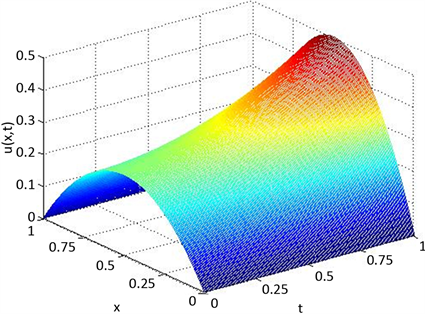Figure 1. Exact surface solutionFigure 2. Universal difference scheme for surface solution when θ = 0.2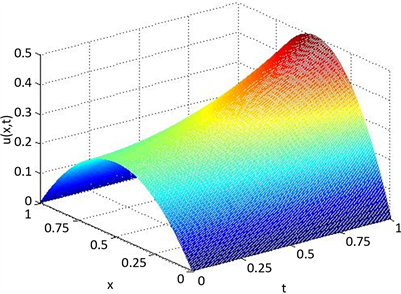Figure 3. Universal difference scheme for surface solution when θ = 0.4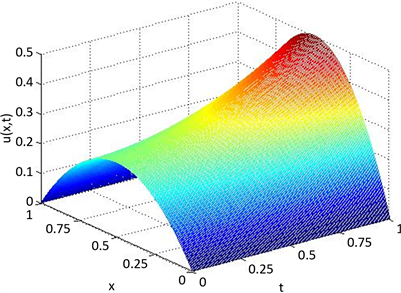Figure 4. Universal difference scheme for surface solution when θ = 0.5Table 1. Error analysis of solutions of universal difference schemes (M = 200, N = 100)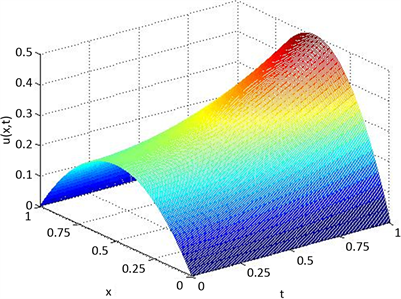Figure 5. Solution of C-N (θ = 0.5) format at N = 200 and M = 50Figure 6. Solution of C-N (θ = 0.5) format at N = 400 and M = 200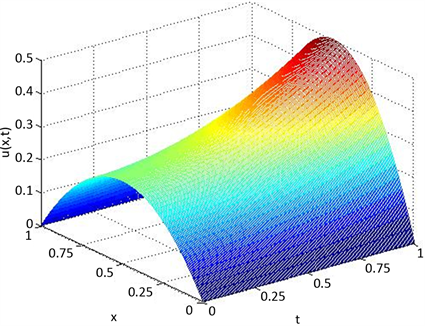Figure 7. Implicit (θ = 1) scheme solutions for N = 200 and M = 50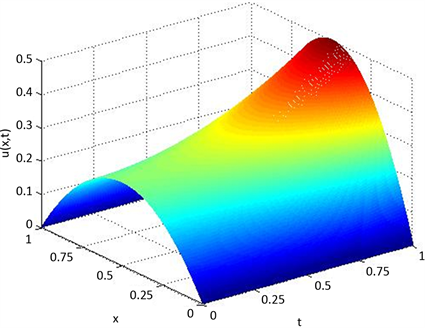Figure 8. Implicit (θ = 1) scheme solutions for N = 400 and M = 200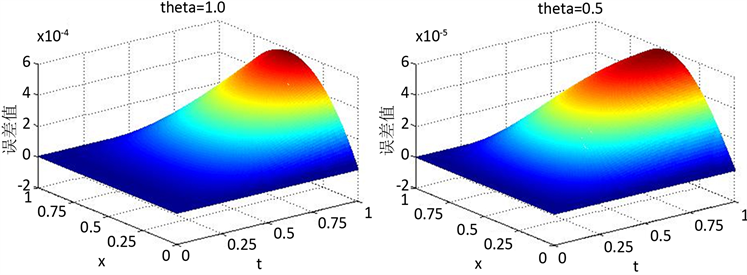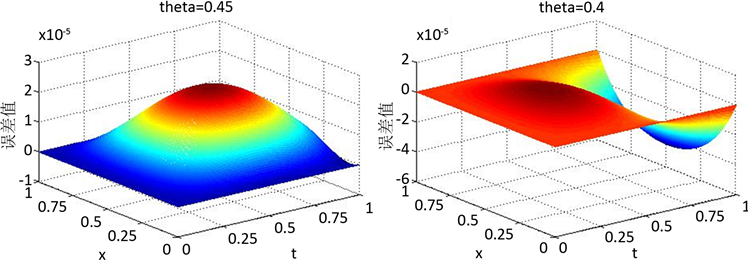Figure 9. Error surface of universal difference schemes when theta takes different values

5. 结论

A Kind of Efficient Universal Differential Methods for Fractional Telegraph Equations[J]. 应用数学进展, 2019, 08(09): 1544-1555. https://doi.org/10.12677/AAM.2019.89181

1. 1. 郭柏林, 蒲学科, 黄凤辉. 分数阶偏微分方程及其数值解[M]. 北京: 科学出版社, 2001.

2. 2. 周激流. 分数阶微积分原理及其在现代信号分析与处理中的应用[M]. 北京: 科学出版社, 2010.

3. 3. 孙志忠, 高广花. 分数阶微分方程的有限差分方法[M]. 北京: 科学出版社, 2015.

4. 4. 刘发旺, 庄平辉, 刘青霞. 分数阶偏微分方程数值方法及其应用[M]. 北京:科学出版社, 2015.

5. 5. 章红梅, 刘发旺. 时间分数阶电报方程的一种解技巧[J]. 厦门大学学报: 自然科学版, 2006, 46(1): 10-13.

6. 6. Chen, J., Liu, F. and Anh, V. (2008) Analytical Solution for the Time-Fractional Telegraph Equation by the Method of Separating Variables. Journal of Mathematical Analysis and Applications, 338, 1364-1377.

7. 7. 王学彬, 刘发旺. 二维和三维的时间分数阶电报方程的解析解[J]. 山东大学学报(理学版), 2012, 47(8): 114-121.

8. 8. 王学彬, 刘发旺. Dirich1et边界条件下时间分数阶电报方程的解析解[J]. 山东大学学报: 自然科学版, 2012, 47(8): 1-9.

9. 9. Camargo, R.F., Chiacchio, A.O. and Oliveira, E.C. (2008) Dif-ferentiation to Fractional Orders and the Fractional Telegraph Equation. Journal of Mathematical Physics, 49, Article ID: 033505. https://doi.org/10.1063/1.2890375

10. 10. Ford, N.J., Rodrigues, M.M., Xiao, J. and Yan, Y. (2013) Numerical Analysis of a Two-Parameter Fractional Telegraph Equation. Journal of Computational and Applied Mathematics, 249, 95-106. https://doi.org/10.1016/j.cam.2013.02.009

11. 11. Saadatmandi, A. and Mohabbati, M. (2015) Numerical Solution of Fractional Telegraph Equation via the Tau Method. Mathematical Reports, 17, 155-166.

12. 12. Kumar, S. (2014) A New Analytical Modelling for Fractional Telegraph Equation via Laplace Transform. Applied Mathematical Modelling, 38, 3154-3163. https://doi.org/10.1016/j.apm.2013.11.035

13. 13. 牛变玲, 李灯熬, 赵富强, 解加全. 分数阶电报方程的Chebyshev多项式数值解法研究[J]. 工程数学学报, 2018, 35(1): 79-87.

14. 14. 陈善镇. 两类空间分数阶偏微分方程模型有限差分逼近的若干研究[D]: [博士学位论文]. 济南: 山东大学数学学院, 2015.